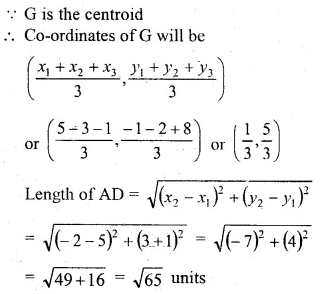# ML Aggarwal Class 10 Solutions for ICSE Maths Chapter 11 Section Formula Chapter Test

## ML Aggarwal Class 10 Solutions for ICSE Maths Chapter 11 Section Formula Chapter Test

ML Aggarwal Class 10 Solutions for ICSE Maths Chapter 11 Section Formula Chapter Test

Question 1.
The base BC of an equilateral triangle ABC lies on y-axis. The coordinates of point C are (0, -3). If the origin is the mid-point of the base BC, find the coordinates of the points A and B
Solution:Question 2.
A and B have co-ordinates (4, 3) and (0, 1), Find
(i) the image A’ of A under reflection in the y-axis.
(ii) the image of B’ of B under reflection in the line AA’.
(iii) the length of A’B’.
Solution: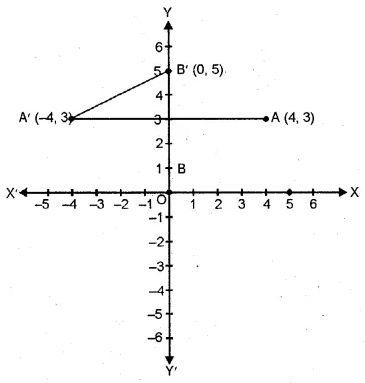Question 3.
Find the co-ordinates of the point that divides the line segment joining the points P (5, -2) and Q (9, 6) internally in the ratio of 3 : 1.
Solution:Question 4.
Find the coordinates of the point P which is three-fourths of the way from A (3, 1) to B (-2, 5).
Solution:Question 5.
P and Q are the points on the line segment joining the points A (3, -1) and B (-6, 5) such that AP = PQ = QB. Find the co-ordinates of P and Q.
Solution: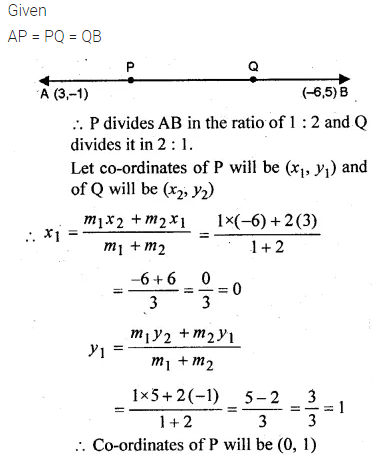Question 6.
The centre of a circle is (α + 2, α – 5). Find the value of a given that the circle passes through the points (2, -2) and (8, -2).
Solution:Question 7.
The mid-point of the line joining A (2, p) and B (q, 4) is (3, 5). Calculate the numerical values of p and q.
Solution:Question 8.
The ends of a diameter of a circle have the co-ordinates (3, 0) and (-5, 6). PQ is another diameter where Q has the coordinates ( -1, -2). Find the co-ordinates of P and the radius of the circle.
Solution:Question 9.
In what ratio does the point (-4, 6) divide the line segment joining the points A(-6, 10) and B (3, -8)?
Solution:Question 10.
Find the ratio in which the point P (-3, p) divides the line segment joining the points (-5, -4) and (-2, 3). Hence find the value of p.
Solution:Question 11.
In what ratio is the line joining the points (4, 2) and (3, -5) divided by the x-axis? Also, find the co-ordinates of the point of division.
Solution:Question 12.
If the abscissa of a point P is 2, find the ratio in which it divides the line segment joining the points (-4, -3) and (6, 3). Hence, find the co-ordinates of P.
Solution:Question 13.
Determine the ratio in which the line 2x + y – 4 = 0 divide the line segment joining the points A (2, -2) and B (3, 7). Also, find the co-ordinates of the point of division.
Solution: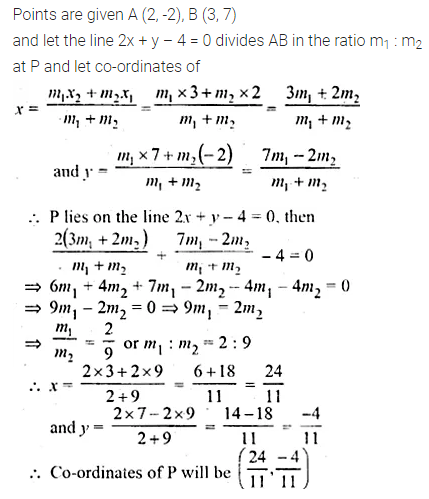Question 14.
Point A(2, -3) is reflected in the v-axis onto point A’. Then the point A’ is reflected in the line x = 4 onto the point A”.
(i) Write the coordinates of A’ and A”.
(ii) Find the ratio in which the line segment AA” is divided by the x-axis. Also, find the coordinates of the point of division.
Solution:Question 15.
ABCD is a parallelogram. If the coordinates of A, B and D are (10, -6), (2, -6) and (4, -2) respectively, find the co-ordinates of C.
Solution:Question 16.
ABCD is a parallelogram whose vertices A and B have co-ordinates (2, -3) and (-1, -1) respectively. If the diagonals of the parallelogram meet at the point M(1, -4), find the co-ordinates of C and D. Hence, find the perimeter of the parallelogram. find the perimeter of the parallelogram.
Solution: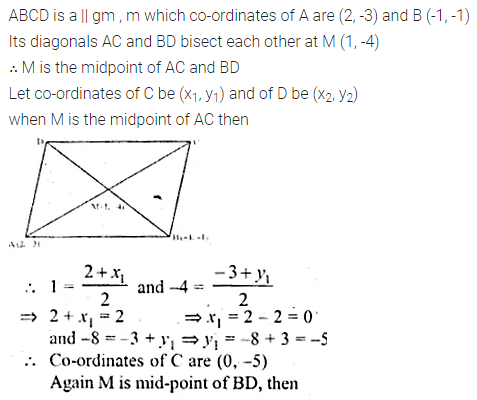Question 17.
In the adjoining figure, P (3, 1) is the point on the line segment AB such that AP : PB = 2 : 3. Find the co-ordinates of A and B.Solution:Question 18.
Given, O, (0, 0), P(1, 2), S(-3, 0) P divides OQ in the ratio of 2 : 3 and OPRS is a parallelogram.
Find : (i) the co-ordinates of Q.
(ii)the co-ordinates of R.
(iii) the ratio in which RQ is divided by y-axis.Solution:Question 19.
If A (5, -1), B (-3, -2) and C (-1, 8) are the vertices of a triangle ABC, find the length of the median through A and the co-ordinates of the centroid of triangle ABC.
Solution: